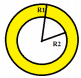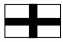Courses

# Mensuration - MCQ 4

## 20 Questions MCQ Test Quantitative Aptitude for Competitive Examinations | Mensuration - MCQ 4

Description
This mock test of Mensuration - MCQ 4 for Quant helps you for every Quant entrance exam. This contains 20 Multiple Choice Questions for Quant Mensuration - MCQ 4 (mcq) to study with solutions a complete question bank. The solved questions answers in this Mensuration - MCQ 4 quiz give you a good mix of easy questions and tough questions. Quant students definitely take this Mensuration - MCQ 4 exercise for a better result in the exam. You can find other Mensuration - MCQ 4 extra questions, long questions & short questions for Quant on EduRev as well by searching above.
QUESTION: 1

### A circular wire of radius 56 cm is cut and bent in the form of a rectangle whose sides are in the ratio of 6:5. The smaller side of the rectangle is

Solution:

The perimeter of the circle, that is, the rectangle is,
P=2πr = 2 * 22/7 * 56 =16×22 cm.
Let us assume the actual length and breadth of the rectangle be, 6xand 5x
So perimeter will be,
P=2(6x+5x)=22x
16×22=22x
X=16.
The smaller side or breadth =5x=80cm

QUESTION: 2

### The circumference of a circle is twice the perimeter of a rectangle. The area of the circle is 5544sq cm. What is the area of the rectangle if the length of the rectangle is 30cm ?

Solution:

Area of circle = (22/7)*r2
5544 = (22/7)*r2
r2 = 5544*7/22
r = 42
circumference of the circle = 2*(22/7)*42 = 264cm
perimeter of the rectangle = 2(30+b)
264/2 = 60+2b
132 = 60+2b
2b = 72
b = 36cm
Area of the rectangle = 36*30 = 1080sq

QUESTION: 3

### The ratio between the perimeter and the breadth of a rectangle is 5:1. If the area of the rectangle is 294 square cm, what is the length of the rectangle?

Solution:

Perimeter=2*(l+b)
2*(l+b)/b = 5/1
2l+2b = 5b
l= 3/2 b.
Area =l*b= 294 sq. units.
3/2 * b2 = 294
b=14
l= 21

QUESTION: 4

A rectangular sheet of metal is 40cm by 15cm. Equal squares of side 5cm are cut off at the corners and the remainder is folded up to form an open rectangular box. The volume of the box is

Solution:

Length of rectangular sheet = 40 cm
Breadth of rectangular sheet = 15 cm
Since, squares of side 5 cm are cut off from the corner of the sheet.
Therefore, new length = 40 – (5 + 5) cm = 30 cm
new breadth = 15 – (5 + 5) cm = 5 cm
Now, volume of the open box = (30 × 5 × 5) cm3 = 750 cm3

QUESTION: 5

10 cylindrical pillars of a building have to be painted. The diameter of each pillar is 70 cm and the height is 4m. What is the cost of painting at the rate of Rs. 10 per square metre?

Solution:

CSA of 10 cyc= 10*2πrh
= 2 * 22/7 * 35/100 * 4
= 44*35*4/(700)
= 10*176*35/700
= 176*35 /70
Cost = 10*176*35/70
= 880rs

QUESTION: 6

In a rectangle the ratio of the length and breadth is 3:2. If each of the length and breadth is increased by 4m their ratio becomes 10:7. The area of the original rectangle in m² is?

Solution:

[3x + 4 / 2x + 4] = 10/ 7
x = 12
Area of the original rectangle = 3x * 2x = 6x²
Area of the original rectangle = 6 * 144 = 864 m²

QUESTION: 7

The length of a rectangular plot is 10 metres more than its breadth. If the cost of fencing the plot @ 26.50 per metre is Rs. 5300, what is the length of the plot in metres?

Solution:

Then, length = (x + 20) metres.
Perimeter = 5300/26.50 = 200m
2[(x + 10) + x] = 200
x = 45.
Hence, length = x + 10 = 55 m.

QUESTION: 8

A tank is 25 m long, 12 m wide and 6 m deep. The cost of plastering its walls and bottom at 25paise per sq. m, is:

Solution:

Area to be plastered = [2(l + b) x h] + (l x b)
Area= {[2(25 + 12) x 6] + (25 x 12)} = 744 m2
Cost of plastering = 744 * 25/100 = 186

QUESTION: 9

A rectangular field is to be fenced on three sides leaving a side of 30 feet uncovered. If the area of the field is 720 sq. feet, how many feet of fencing will be required?

Solution:

L = 30; lb = 720;
B= 24 ft
Length of fencing = l + 2b = 30 + 48 = 78 ft

QUESTION: 10

The perimeter of a rectangular field is 120 m and the difference between its two adjacent sides is 40 m. The sides of the square field whose area is equal to this rectangular field is?

Solution:

Perimeter of rectangle = 2(l + b) = 120
l + b = 60m — (1)
l – b = 40m –(2)
From (1) and (2)
l = 50 m; b = 10m
Area of rectangle = 500m² = Area of Square
Side of a square = 10√5

QUESTION: 11

If the area of a square is equal to the area of that rectangle whose width is double of the one side of the square then the ratio of the length to the breadth of the rectangle will be?

Solution:

b = 2a
a = b/2
Area of square = b²/4 = Area of rectangle
l * b = b²/4 ⇒ l = b/4
l / b =(b/4)/b ⇒ 1:4

QUESTION: 12

In a rectangle the ratio of the length and breadth is 3:2. If each of the length and breadth is increased by 3m their ratio becomes 10:7. The area of the original rectangle in m² is?

Solution:

[3x + 3 / 2x + 3] = 10/ 7
x = 9
Area of the original rectangle = 3x * 2x = 6x²
Area of the original rectangle = 6 * 81 = 486m²

QUESTION: 13

The perimeter of a rectangular field is 120 m and the difference between its two adjacent sides is 30 m. The sides of the square field whose area is equal to this rectangular field is?

Solution:

Perimeter of rectangle = 2(l + b) = 120
l + b = 60m — (1)
l – b = 30m –(2)
From (1) and (2)
l = 45 m; b = 15m
Area of rectangle = 675m² = Area of Square
Side of a square = 15√3

QUESTION: 14

If each side pair of opposite sides of a square is increased by 10m, the ratio of the length and breadth of the rectangular so formed becomes 5:3. The area of the old square is?

Solution:

(x+10) / x = 5 / 3
3x + 30 = 5x
x = 15m; Area = 225m²

QUESTION: 15

The area of the garden formed by two concentric circles with circumferences 44m and 176 m respectively is?

Solution:2πR1 = 176
R1 = 28m
2πR2 = 44
R2 = 7m
Area of the garden = π(R1² – R2²) = 22/7(784 – 49) =2310m²

QUESTION: 16

One of the adjacent sides of a rectangular courtyard is 5m and its diagonal measures 13 m long. What is the area of the courtyard?

Solution:

Another side = √[(13)² – (5)²] = 12m
Area = 12 * 5 = 60m²

QUESTION: 17

The length of a park is four times of its breadth. A playground whose area is 1600 m² covers 1/4th part of the park. The length of the park is?

Solution:

l = 4b
Area of the park = 4 * 1600 = 6400m²
l * b = 6400
l * l/4 = 6400
l² = 6400 * 4; l = 80 * 2 = 160 m

QUESTION: 18

Two roads each 10m wide has been made running perpendicularly to each other inside a rectangular field of dimension 90m X 50m. What is the cost of spreading pebbles over them at the rate of Rs.8 per m².?

Solution:Area of Roads = (l + b – w) * w
Area of Roads = (90 + 50 – 10) * 10 = 1300m²
Cost = 1300 * 8 = 10400

QUESTION: 19

The width of a rectangular piece of land is 1/3 rd of its length. If the perimeter of the piece of land is 320m its length is?

Solution:

length = l ; breadth = l/3
2(l + b) = 320
2(l + l/3) = 320
l = 320 * 3/8 = 120m

QUESTION: 20

The perimeter of a square and a rectangle are equal. If the length of rectangle is 24m and breadth of the rectangle is 1/3 rd of its length, then the area of the square will be?

Solution:

Perimeter of square = Perimeter of rectangle
4a = 2(24 + 8)
a = 16
Area = 256m²